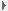Home About Journal Editorial Board Instruction Subscriptions Contacts Us 中文2011, Vol. 43Issue (04) : 462-472     DOI:|
 Modeling Language Components in Mathematical Items Using Multiple Random Effects IRT Models
YANG Xiang-Dong;CHIU Lorraine;WEI Yun
(1 Institute of Curriculum and Instruction, East China Normal University, Shanghai 200062, China)
(2 Department of Psychological Research in Education, University of Kansas, USA)
 Download: PDF(475 KB)   Export: BibTeX | EndNote | Reference Manager | ProCite | RefWorks
 Abstract  Language components in mathematical word problems may have profound impacts on the problem-solving processes engaged by examinees. When a math problem is presented by means of language statements, translation of these language statements into mathematical propositions must be carried out properly before relevant information can be integrated into a coherent representation of the problem. As many studies have shown, the impacts of such language components are unlikely to occur uniformly across examinees. Yet existing approaches that address this issue focus only on (1) examining the first-order correlation between the reading and mathematical abilities of examinees, (2) identifying a general language factor through factor analysis, and (3) examining the average impacts of specific language features on math item properties through linear regression or linear logistic test model (LLTM). While the first two approaches fall short of providing targeted information about the specific aspects of language components that impact examinees’ mathematical problem solving, the third approach is based on an unrealistic assumption of constant impacts of such language components. Therefore, an alternative approach needs to be formulated in order to model individual-specific impacts of particular language features on math item proficiency. The current study applies item response theory (IRT) models with multiple random effects to investigate the effects of language components in mathematical items on examinees’ performances. This approach starts by studying relevant literature and searching for a cognitive processing model for mathematical problem solving, and then identifies specific item stimulus features in the problem statements to represent the language components according to the model. By encoding items in a third-grade mathematical test according to the set of identified stimulus features, a set of models that operationalize different cognitive principles are then applied to the data obtained from the test. This approach provides a rigorous method for examining not only the average impacts of specific language features on item properties over the sample, but also variations of such impacts among examinees. Consequently, it allows researchers to investigate the interactions between the characteristics of a mathematical problem and the cognitive abilities of a particular examinee. Results from this study show that, among the six item stimulus features that were identified based on Mayer’s cognitive theory of mathematical problem solving and its associated literature, all but one significantly affect the difficulty of mathematical items. Difficult items tend to have more words, feature a higher proposition density, and require the examinee to either translate information from a graph or table or to generate mathematical equations from the problem statements. In addition to the variation of mathematical ability across examinees, proposition density of the mathematical items shows differentiated impacts for different examinees in the testing sample. While the random effects of proposition density inversely relate to the mathematical abilities of examinees in general, proposition density exerts more impacts on the math problem solving for examinees with low abilities, and such impact is diminished as the examinee’s ability increases. Corresponding Authors: YANG Xiang-Dong Issue Date: 30 April 2011
 ServiceE-mail this articleE-mail AlertRSS Articles by authorsYANG Xiang-DongCHIU LorraineWEI Yun
 Cite this article: YANG Xiang-Dong,CHIU Lorraine,WEI Yun. Modeling Language Components in Mathematical Items Using Multiple Random Effects IRT Models[J]. , 2011, 43(04): 462-472. URL: http://journal.psych.ac.cn/xlxb/EN/      OR     http://journal.psych.ac.cn/xlxb/EN/Y2011/V43/I04/462
 No related articles found!
Viewed
Full text

Abstract

Cited

Shared
Discussed